What's New in Macro Processing

Macro Processing - called from 'Equilib > Reactants Window > File > Macro processing > Run macro ...'

What follows are summaries of the more important programming changes in Macro Processing that have appeared in:

History

Macro Processing (MP) was initially developed for FactSage beta testing and quality control. Whenever a database was edited or a program (mainly Equilib) modified, it was necessary to verify all the old calculations still worked as before. With MP one can easily perform a limitless number of standard calculations and numerically compare old and new log files. MP is particularly useful in database development where all the standard phase diagrams for a given database can be re-plotted and compared with the previous plots.

Some databases (for example, SGTE) required almost one day to regenerate all the phase diagrams. The improvements in recent years to the ChemApp Gibbs energy minimizer algorithm and the modern Fortran compiler (Intel(R) Visual Fortran Compiler 17) have drastically reduced the computational time. MP really saves the database developer a lot of time. It has been invaluable in implementing the new solution database structure.

MP was not designed for public use - hence the command structure can be a challenge. MP was made public in FactSage 5.1 (2002). Since then we have tried to make MP user-friendly (i.e. less user hostile) but it is a losing battle. However the Notepad++ text editor that accesses the FactSageMacros.xml defined language (see FactSage 7.3) should help.

FactSage 5.5 (2007)

A new type of variable called a 'macro \$function()' has been introduced. Macro \$functions have arguments contained within the parentheses, (). The arguments are separated by spaces or commas and may be a %variable, a thermochemical \$variable\$, but not another \$function. A line in the macro file may have several \$functions. The new functions include:
• String manipulation - \$LCASE, \$UCASE, \$LEFT, \$RIGHT, \$MID
• Output formatting - \$FORMATE, \$FORMATF, \$FORMATG, \$FORMATI
• Mathematical functions - \$ABS, \$EXP, \$LN, \$LOG10, \$FIX, \$INT, \$RND, \$RNDINT, \$MIN, \$MAX, \$MINI, \$MAXI, \$MEAN
• Mixed mathematical expressions - \$MATH. This versatile function enables you to enter a mixed expression that includes the operators, + - / * ^ \, as well the common functions abs(), ln(), log10(), exp(), cos(), sin(), tan(), acos(), asin(), atan(), fix(), int(), sqr(), cbr() and fact().
Examples:     \$MATH(cos(100)^2 + sin(100)^2) => 1.     \$MATH((5!-(2-5)^2)/(sqr(abs(2^4-3^5))) => 7.36732...
• Other functions - \$EXIST, \$CONSTANT

New SET variables have been added, including:
• SET PAGE 'value' - to select a particular page from many pages of results
• SET REACTANT ADD - to add a new reactant, or DEL to delete (remove) an existing one
• SET SELECT GAS (LIQUID AQUEOUS SOLID COMPOUND) + (-!) ['data' ['species']] - to include (or exclude) compound species in the equilibrium calculation
• SET SELECT SOLUTION + (-!IJ) 'data-phase' - to include (or exclude) solution phases in the equilibrium calculation
Other variables and commands have been added, including:
• RUNMACRO 'filename.mac' - to quit the current macro and run filename.mac
• SHELLEXECUTE 'filename' - to open a file using the Windows default application (NotePad, WordPad, Excel ...)
• thermochemical \$variables\$ for solution integral and partial properties; component lists and amounts; and more.
The Macro Processing Manual has been updated to include the new variables. New *.mac files have been added to the Macro directory of examples:
- an example of the new \$functions is given in 'EquiEx_List_Functions.mac'.
- an example of most thermochemical variables is given in 'EquiEx_List_Thermo.mac'.
- an example of SET SELECT is given in 'EquiEx_Set_Variables.mac'.

FactSage 6.3 (2012)

DOCASE

The CASE statements within the DOCASE ... ENDDO commands now include a test for a range ('value1' TO 'value2') and a logical condition test (IS 'operator < > <> >= or <=' 'value').

```DO CASE %1
CASE 0 1 2       // If %1 has the value 0, 1 or 2
(macro lines)
CASE 3 TO 50.5   // If %1 is in the range 3 to 50.5
(macro lines)
CASE IS <= -100  // If %1 is less than or equal to -100
(macro lines)
CASE IS <> 1000  // If %1 is not equal to 1000
(macro lines)
CASE ELSE
(macro lines)
ENDDO
```

New \$Variable\$s

New variables have been introduced into 6.3 macro processing.

```\$TEMPDIR\ = temporary directory - environment variable defined by Windows

Extensive Property Changes:
\$E_DOK\$  = 'OK' when delta values calculated (e.g. initial conditions defined)
\$E_DC\$  = Delta Cp heat capacity
\$E_DF\$  = Delta Gibbs Free Energy
\$E_DG\$  = Delta Gibbs Free Energy
\$E_DH\$  = Delta Enthalpy
\$E_DS\$  = Delta Entropy
\$E_DV\$  = Delta Volume

Input Reactant Values:
\$R_SOK\$  = 'OK' when states are known  (e.g. initial conditions defined)
\$R_p*\$ = phase state (1=gas, 2=liquid, 3=aqueous, 4=solid, 5=solution) of reactant *
\$R_s*\$ = short state (g, l, aq, s, s2) of reactant *
\$R_S*\$ = full state (gas, liquid, aqueous, solid, solid-2) of reactant *

Chemical and Physical Properties of Solution *:

\$E_CP*_Evolts\$          = aqueous Eh
\$E_CP*_pH\$              = aqueous pH
\$E_CP*_total_molality\$  = total solute molality
\$E_CP*_ionic_strength\$  = ionc strength
\$E_CP*_osmotic_coeff\$   = osmotic coefficient
\$E_CP*_magnetic_moment\$ = magnetic moment
\$E_CP*_Curie_T\$         = Curie temperature
\$E_CP*_viscosity\$       = viscosity
\$E_CP*_surface_tension\$ = surface tension
\$E_CP*_thermal_cond\$    = thermal conductivity
\$E_CP*_electrical_cond\$ = electrical conductivity
\$E_CP*_surface_energy\$  = surface energy
\$E_CP*_emissivity\$      = emissivity
\$E_CP*_density\$         = density
\$E_CP*_thermal_expan\$   = thermal_expansivity
\$E_CP*_bulk_modulus\$    = bulk_modulus
\$E_CP*_Grueneisen\$      = Grueneisen
\$E_CP*_a_lattice\$       = lattice parameter a
\$E_CP*_c_lattice\$       = lattice parameter c
```
Many of new variables and commands are used in the updated macro file EquiEx_List_Results.mac located in the macros folder.

SET OUTPUT

New output format commands for the display in the Results Window

```
SET OUTPUT FORMAT FACT CHEMSAGE  // FACT and then ChemSage format
SET OUTPUT FORMAT CHEMSAGE FACT  // ChemSage and then FACT format
SET OUTPUT FORMAT FACT           // FACT format only
SET OUTPUT FORMAT FACT CHEMSAGE  // ChemSage format only
```

SET SELECT

In FactSage 6.2 problems were encoutered with phase and species selection 'SET SELECT' commands of the type:

```SET SELECT COMPOUND + (or - !) [NICK [SPECIES]]
SET SELECT SOLUTION + (or - !) [NICK-Phase [SPECIES]]
SET SELECT GAS (or LIQUID AQUEOUS SOLID) + (or - !) [NICK [SPECIES]]
```
In FactSage 6.3 these problems have been fixed and the SELECT commands thoroughly tested.

FactSage 6.4 (2013)

All the macro files stored in the /Macro folder have been checked with FactSage 6.4 and the files updated modifications where necessary.

If you unfamiliar with macro processing and want to try it go to the Reactants Window, click on 'File > Macro processing > Run macro > Macros Directory ...' and select EquiEx_SET_Variables.mac.

If you want to see the information generated (but hidden) when you load and run one of your saved Equi*.dat files then select EquiEx_List_Results.mac and specify the Equi*.dat file.

For an example of how to open and interact (read and write) with an Excel spreadsheet (.Xls file) and how to follow the progress of equilibrium calculations in real time then select EQUIEx_MgCl2_O2_F2.mac.

New \$Variable\$s

New variables have been introduced into 6.4 macro processing.

```Extensive Property Changes: \$E_D*\$, * = OK, C, F, G, H, S, V

\$E_DOK\$ = 'OK' when delta values calculated (e.g. initial conditions defined)
\$E_DC\$  = Delta Cp heat capacity
\$E_DF\$  = Delta Gibbs free energy
\$E_DG\$  = Delta Gibbs free energy
\$E_DH\$  = Delta enthalpy
\$E_DS\$  = Delta entropy
\$E_DV\$  = Delta volume

Database search options \$DS_*\$, * = GION, AQUA, CXHY, MINS

\$DS_GION\$ : 0 = exclude gas ions, 1 = include gas ions
\$DS_AQUA\$ : 0 = exclude aqueous phase, 1 = include aqueous phase
\$DS_CXHY\$ : 1 to 99 = maximum value of X in organic molecules CxHy..
\$DS_MINS\$ : 1 = minimum 1 component solutions, 2 = minimum 2 component solutions,

Databases: Compound \$DB_C_*\$ and Solution \$DB_S_*\$ values, ? = C or S,  * = 1 - 48

\$DB_?_M\$  : number of selected compound (\$DB_C_M\$) or solution (\$DB_S_M\$) databases
\$DB_?_F*\$ : file name of database *
\$DB_?_P*\$ : path and file name of database *
\$DB_?_N*\$ : nick name of database *
\$DB_?_I*\$ : information about database *
\$DB_?_V*\$ : database version *
\$DB_?_T*\$ : time and date stamp of database file *
```

SET SELECT

New data search commands have neen added - SET DATASEARCH GION (NOGION AQUA NOAQUA CXHY MINS1)

```SET DATASEARCH GION (NOGION)     : include (exclude) gaseous ions (plasmas)
SET DATASEARCH AQUA (NOAQUA)     : include (exclude) aqueous species
SET DATASEARCH CXHY 'value 1-99' : CxHy .. maximum value of x in organic molecules
SET DATASEARCH MINS1 (MINS2)     : minimum number of solution components is 1 (2)
```
SET ESTIMATE

New commands for redefining the estimatete of final property have been added - SET ESTIMATE T (P V ALPHA)

```SET ESTIMATE T 'value'     : redefine the estimate of final T
SET ESTIMATE P 'value'     : redefine the estimate of final P
SET ESTIMATE V 'value'     : redefine the estimate of final V
SET ESTIMATE ALPHA 'value' : redefine the estimate of final ALPHA
```
Examples of the new variables and commands can be found in the updated macro files EquiEx_SET_Variables.mac and EquiEx_List_Results.mac located in the macros folder.

OLE Commands for Excel Worksheets

The number of OLE (Object Linking and Embedding) commands for Excel Worksheets has been expanded. It is now possible to open both *.xlsx type files in addition to *.xls. New CLOSE and QUIT commands enable you to save and close the Excel link. There are new options to dynamically define cell size and text font, color, alignment, etc. in the spreadsheet. Examples of the new commands are presented in EquiEx_Xls_Simple_IO.mac which has been rewritten for FactSage 6.4 and is located in the Macros Directory.

```
OLEn CLOSE                       // close the Worksheet; Excel will prompt you to save
OLEn CLOSE SAVE                  // close and save the Worksheet without prompting
OLEn CLOSE SAVE 'ExcelFileName'  // close and save in a *.xls or *.xlsx file
OLEn QUIT                        // close the Worksheet without saving

OLEn CELLS     ALL   'options'   // apply options to all cells in the Worksheet
OLEn COLUMNS  'F:F'  'options'   // apply options to column F
OLEn ROWS     '2:3'  'options'   // apply options to rows 2 and 3
OLEn RANGE  'B1:C4'  'options'   // apply options to a range
OLEn NAME    'NAME'  'options'   // apply options to a range name

List of 'options' for OLEn CELLS (COLUMNS ROWS RANGE NAME):

AUTOFIT                  // autofit column width
// ex: OLE1 CELLS ALL AUTOFIT autofits all cells in the Worksheet
BOLD TRUE (FALSE)        // Bold text - TRUE is default.   BOLD may be replaced by:
// ITALIC, SHADOW, STRIKETHROUGH, SUBSCRIPT, SUPERSCRIPT or UNDERLINE
// ex: OLE1 ROWS 2:3 BOLD
CENTER (LEFT RIGHT)      // alignment of contents in each cell
CLEAR                    // clear the contents in each cell
COLOR 'color'            // text color where 'color' is one of  BLACK, MAROON, GREEN,
// OLIVE, NAVY, PURPLE, TEAL, GRAY, SILVER, RED, LIME, YELLOW, BLUE, FUSHIA, AQUA or WHITE
COLOR RGB(i,j,k)         // RGB color where i, j and k in the range 0 - 255
// ex: OLE1 RANGE A1:C:4 COLOR RGB(0,0,255)   - same as 'COLOR BLUE'
FONTSTYLE 'font style'   // font style e.g. 'Bold_Italic' - use _ for spaces
HEIGHT 'value'           // set rows to a common height
SIZE   'value'           // set font size to a common value
WIDTH  'value'           // set columns to a common width
```

FactSage 7.0 (2015)

All the macro files stored in the /Macro folder have been checked with FactSage 7.0 and the files updated where necessary.

If you unfamiliar with macro processing go to the Reactants Window, click on 'File > Macro processing > Run macro > Macros Directory ...' and select EquiEx_SET_Variables.mac.

If you want to see the hidden generated information when you load and run one of your saved Equi*.dat files then select EquiEx_List_Results.mac and specify the Equi*.dat file.

For an example of how to open and interact (read and write) with an Excel Worksheet (.Xls and .xlsx files) and a Text Spreadsheet then select EquiEx_Xls_Simple_IO.mac.

For a complete list of examples click on 'File > Macro processing > Macro Processing - Examples ...'.

1. New Organisation and Documentation

 The Macro Processing program has been reorganised, terminology has been simplified, new commands and functions have been introduced, and new documents have been added. Examples of the new commands and functions are given in the macro EquiEx_New_in_7.0.mac. The system \$Variables have been integrated into \$Functions. This has no effect on the macro files. Compressed multi-word terminology for commands and functions such as SHELLEXECUTE SHOWLASTPD \$FILESHORTDIR etc. have been expanded into SHELL_EXECUTE SHOW_LAST_PD \$FILE_SHORT_DIR etc. in order to improve readability. The changes are backward compatible - i.e. the old terminology still works. A new document, Macro Processing - Summary, has been added to the menu. This summarizes all the macro variables, functions and commands that are available in macro processing. A new document, Macro Processing - Examples, has been added to the menu. This lists the examples and introduces all the Equilib macro files (EquiEx*.mac) that are stored in the Macros folder (typically 'c:\FactSage\Macros\').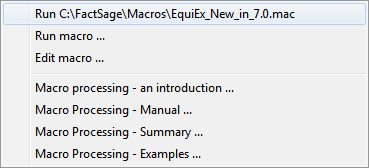2. New Commands

 2.1 DEBUG The new command DEBUG /ON activates the debug mode and displays the DEBUG Macro Window which lists the current values of all user-defined %variables. Each time a DEBUG command is issued the DEBUG Macro Window is updated with the current values. It is hoped that this will become a valuable tool in macro processing development. As an example, load and run the macro file EquiEx_New_in_7.0.mac that is stored in the Macros folder. ``` DEBUG /ON // Activates the DEBUG Macro Window DEBUG /OFF // Deactivates the debug mode // - this is the default setting. DEBUG 'caption' // Lists current values of all %VARIABLES //- 'caption' is the display heading DEBUG /DEL %var1 %var2 ... // Drops %VARIABLES from the debug list // The DEBUG commands are a debugging aid // and have no effect on the macro processing. ``` The screenshot shows the 'DEBUG Macro Window' that is displayed by the macro EquiEx_New_in_7.0.mac.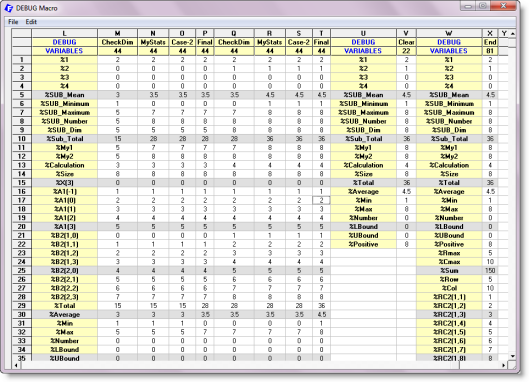2.2 DIM, REDIM

DIM is new in 7.0 and is identical to VARIABLE. It is now possible to declare a 2-dimensional array using DIM (or VARIABLE).

If a variable array has are already been declared, it can be re-dimensioned by using the new REDIM.

```
DIM %A %B(4) %C(2,3) // Declares %A, %B(1) %B(2) %B(3) %B(4),
// %C(1,1) %C(1,2) %C(1,3) %C(2,1) %C(2,2) %C(2,3)
REDIM %A(0:2,2)      // Re-dimensions %A as %A(0,1) %A(0,2) %A(1,1) %A(1,2) %A(2,1) %A(2,2)
```

2.3 Defining tabular values

```
%total = \$E_sMs\$  //  total number of product species
DIM %mole(%total) %activity(%total)

// 1. Define the tabular values of activity and moles one at a time
%1 = 1 TO %total
%activity(%1) = \$E_sa%1\$   // or \$E_sa(%1)\$.  Note () aids in the readability.
%mole(%1)     = \$E_sm%1\$   // or \$E_sm(%1)\$
%1 LOOP

// 2. More efficient way:
%activity(1:%total) = \$E_sa(1:%total)\$  // or \$E_sa()\$  or \$E_sa\$
%mole(1:%total)     = \$E_sm(1:%total)\$  // or \$E_sm()\$  or \$E_sm\$

// 3. Simpler way:
%activity()    = \$E_sa()\$  // () is useful since it denotes dimensioned variables.
%mole()        = \$E_sm()\$  //       ...

// 4. Simplest way:
%activity      = \$E_sa\$    // This is OK but without () it is difficult to read or debug.
%mole          = \$E_sm\$    //       ...
```

2.4 Manipulating the definition of thermochemical \$..\$ variables

'(' and ')' can be inserted into the thermochemical \$..\$ variable to improve readability.

For example, in the following lines %act refers to the calculated activity of product species 4 and %gram refers to the number of grams of element (component) 2 in mixture (solution) 3.

```
DIM  %Index1(10) %act %gram
%Index1() = \$ENUM(1) // %Index1(1) = 1 ... %Index1(4) = 4 ... %Index1(10) = 10

%2 = 4
%act = \$E_sa4\$       // activity of product species 4
%act = \$E_sa4:4\$
%act = \$E_sa(4:4)\$
%act = \$E_sa%2\$
%act = \$E_sa(%2)\$
%act = \$E_sa%Index1(4)\$
%act = \$E_sa(%Index1(4))\$
%act = \$E_sa(%Index1(%2))\$
%act = \$E_(sa)((%Index1(%2)))\$

%5 = 2
%6 = 3
%gram = \$E_e2_mg3\$   // grams of element 2 in mixture  3
%gram = \$E_e%5_mg%6\$
%gram = \$E_e2_mg(3)\$
%gram = \$E_e%5_mg(%6)\$
%gram = \$E_e(%Index1(2))_mg(%Index1(3))\$

```
2.5 OLE commands for Text Spreadsheets

An Excel Worksheet is stored in the well-known *.Xls and *Xlsx Spreadsheet files supported by Microsoft. When programming with Excel files there are numerous ways the data stored in the cells of the spreadsheet can be processed, manipulated, plotted etc. By means of Object Linking and Embedding, OLE, you can use an Equilib macro to interact with an Excel Worksheet that may already be open in another application, in which case both applications will be able to share the same work space.

In FactSage 7.0 it is also possible to create an OLE link with a Text Spreadsheet that is stored in a *.txt or *.tab text file. This type of link offers simplicity and speed when compared with the Excel Worksheet. There are often situations where the Text Spreadsheet is preferred to the Excel Worksheet especially in cases where data are simply being posted or manually entered. However data in a Text Spreadsheet cannot be shared with other applications nor can the data be manipulated by the Excel functions.

The Equilib macro processing enables you to create up to 9 simultaneous dynamic links (OLE1 to OLE9) with Excel Worksheets and/or Text Spreadsheets.

All the current OLE commands and options for an Excel Worksheet (e.g. CLOSE, QUIT, CELLS, COLUMNS, ROWS, RANGE, GOTO, AUTOFIT, BOLD TRUE, CENTER, LEFT, RIGHT, CLEAR, COLOR, FONTSTYLE, HEIGHT, SIZE, WIDTH, etc.) also work for the Text Spreadsheet. In a macro to change an Excel Worksheet to a Text Spreadsheet in most cases you only need to replace the initial OLEn declaration as shown next.

```OLEn:
OLEn 'ExcelFileName' ['Sheet-Name']
// Create an OLE link (n = 1 to 9) with an Excel Worksheet stored in an Excel Spreadsheet file (*.xls,*.xlsx).
// ex: OLE1 MyExcel.xlsx  Input  - creates a link to the 'Input' Worksheet of the Excel file.

OLEn 'TextFileName' ['Sheet-Name']
// Create an OLE link (n = 1 to 9) with a Text Spreadsheet stored in an ascii file (*.txt,*.tab).
// ex: OLE1 MyText.txt  Input  - creates a link to the 'Input' Spreadsheet of the Text file.
```
A simple example is given in the macro file EquiEx_Xls_Simple_IO.mac (this example is expanded in FactSage 7) and a more complicated one in EquiEx_CH4-O2-c.mac.

3. New \$Variable functions

The new array functions \$ARRAY_DIM(), \$ARRAY_COUNT(), \$ARRAY_LBOUND() and \$ARRAY_UBOUND():

```
DIM %A %B(4) %C(2,3)
\$ARRAY_DIM(%1)       // => 0 :  %1 is not an array
\$ARRAY_DIM(%B)       // => 1 :  %B() is a 1-dimensional array
\$ARRAY_DIM(%C)       // => 2 :  %C() is a 2-dimensional array
\$ARRAY_COUNT(%B)     // => 4 :  %B has 4 members - %B(1) %B(2) %B(3) %B(4)
\$ARRAY_COUNT(%C)     // => 6 :  %C has 6 members - %C(1,1) %C(1,2) %C(1,3) %C(2,1) %C(2,2) %C(2,3)
\$ARRAY_LBOUND(%B)    // => 1 :  lower limit of dimension - %B(1)
\$ARRAY_LBOUND(%C)    // => 1 :  lower limit of first dimension - %C(1,*)
\$ARRAY_LBOUND(%C 1)  // => 1 :  lower limit of first dimension - %C(1,*)
\$ARRAY_LBOUND(%C 2)  // => 1 :  lower limit of second dimension - %C(*,1)
\$ARRAY_UBOUND(%B)    // => 4 :  upper limit of dimension - %B(4)
\$ARRAY_UBOUND(%C)    // => 2 :  upper limit of first dimension - %C(2,*)
\$ARRAY_UBOUND(%C 1)  // => 2 :  upper limit of first dimension - %C(2,*)
\$ARRAY_UBOUND(%C 2)  // => 3 :  upper limit of second dimension - %C(*,3)
```
The new \$ENUM() function to enumerate the members of a user-defined array - \$ENUM( 'start' ['step'] )
```
DIM %B(4) %C(2,3)
%B() = \$ENUM(1)      // => %B(1) = 1, %B(2) = 2, %B(3) = 3, %B(4) = 1
%B() = \$ENUM(0 -2)   // => %B(1) = 0, %B(2) = -2, %B(3) = -4, %B(4) = -6
%C() = \$ENUM(0)      // => %C(1,1) = 0, %C(1,2) = 1, ... %C(2,2) = 4, %C(2,3) = 5
```
New \$TIME() functions to post the run time -
```
\$TIME                // current time
\$TIME_RUN            // time passed (hh:mm:ss) since the start
\$TIME_RUN_SECS       // time passed (seconds) since the start
\$TIME_INTERVAL       // time passed (hh:mm:ss) since last call to \$TIME_INTERVAL
\$TIME_INTERVAL_SECS  // time passed (seconds) since last call to \$TIME_INTERVAL_SECS
```

4. User-defined \$Functions and Procedures

In FactSage 7.0 it is now possible to program user-defined FUNCTIONS (e.g. \$Function('arguments') and PROCEDURES (e.g. CALL SUBROUTINES) . The FUNCTIONS and PROCEDURES are listed at the end of the macro FUNCTIONS ... END FUNCTIONS and PROCEDURES ... END PROCEDURES) and may be executed from anyway with the macro.

```FUNCTIONS
//  The format of a user-defined function is :
//  FUNCTION \$'function name'('input parameters')
//     - \$'function name name' is any alphanumeric description starting with \$.
//     - 'input parameters' are values passed from the main body.
//  ....macro lines (where 'output variables' are defined)
//  \$'function name' = 'value' (where the value of function is defined)
//  END FUNCTION

FUNCTION \$My-Whatever(arg1 arg2 ...)
... macro lines
\$My-Whatever = 'value'
END FUNCTION

FUNCTION \$My-Another(arg1 arg2 ...)
... macro lines
\$My-Another = 'value'
END FUNCTION

END FUNCTIONS

PROCEDURES
//  The format of a procedure is :
//  'procedure name'('input parameters')
//      - 'procedure name' is any alphanumeric description
//      - 'input parameters' are values are defined in the main body.
//  ... macro lines where 'output variables' are defined)
//  END  'procedure name'

SUB_CheckDim(%A)
.... (macro lines)
END SUB_CheckDim

SUB_Another(%C)
.... (macro lines)
END SUB_Another

END PROCEDURES
```
Examples of user-defined functions and procedures are presented in EquiEx_New_in_7.0.mac and EquiEx_Procedures.mac stored in the Macros folder.

FactSage 7.1 (2017)

All the macro files stored in the Macros folder have been checked with FactSage 7.1 and the files updated where necessary.

If you unfamiliar with macro processing go to the Reactants Window, click on 'File > Macro processing > Run macro > Macros Directory ...' and select EquiEx_SET_Variables.mac.

If you want to see the hidden generated information when you load and run one of your saved Equi*.dat files then select EquiEx_List_Results.mac and specify the Equi*.dat file.

For an example of how to open and interact (read and write) with an Excel Worksheet (.Xls and .xlsx files) and a Text Spreadsheet then select EquiEx_Xls_Simple_IO.mac.

For a complete list of examples click on 'Reactants Window > File > Macro processing > Macro Processing - Examples ...'.

 The Macro Processing Manual, Summary and Examples have been revised to reflect the changes and additions to the program.1. %DIR

The macro command %DIR is used to define the user default directory.

If the macro file is reading and writing various files (.dat, .xls, .txt, etc. ) it is recommended that all the files including the .mac file be stored in the same folder, and it is recommended you should set the value of %DIR to this folder. For example, if the macro file is stored in c:\FactSage\Private\MyMacro.mac then the following line could be inserted at the beginning of the .mac file:

`%DIR = c:\FactSage\Private\ // Directory of the file c:\FactSage\Private\MyMacro.mac `

Alternatively, the following lines are a more general way to define the default location and this does not require you to enter the actual path.

```VARIABLE %Macro
%Macro = \$MACFILE           // Full name of the current macro file - e.g. c:\FactSage\Private\MyMacro.mac
%DIR = \$FILE_DIR(%Macro)    // Set user default directory to the directory of the macro file - e.g. c:\FactSage\Private\
```

2. New Plotting Commands

The new SET PLOT commands mirror the options available in the Equilib Post Processor and Plot Species Selection Windows - 'Equilib Results Window > Output > Plot > Plot Results ...'.

Consult these Post Windows for clarification of the following SET PLOT commands.

As an example, load and run the new macro file EquiEx_CH4-O2_Figure.mac that is stored in the Macros folder.

```
SET PLOT AXIS/CAPTION/LABELS/DISPLAY/SELECT:

SET PLOT AXIS Y-AXIS (X-AXIS) a (n  etc.) Y (log10(Y) ln(Y) ...) 'minimum' 'maximum' 'step'

SET PLOT CAPTION TITLE    'figure title'                 // Figure title
SET PLOT CAPTION SUBTITLE 'figure sub-title'             // and subtitle
SET PLOT CAPTION Y-TITLE (X-TITLE) 'axis title'          // Axes captions
SET PLOT CAPTION LABEL     'X coordinate' 'y coordinate' "label #1"  // User-defined labels
SET PLOT CAPTION LABEL     'X coordinate' 'y coordinate' "label #2"  //
SET PLOT CAPTION LABEL      .... etc.                                //

SET PLOT LABELS  SIZE  2 (2 - 24)           // size of labels  (Post Processor Window)
SET PLOT LABELS  NO    2 (1 - 9)            // number per line
SET PLOT LABELS  CHEMICAL [INTEGER or NONE) // type of label
SET PLOT LABELS  OFFSET       ON (OFF)      // offset labels above the coordinate

SET PLOT DISPLAY COLOR        ON (OFF)      // line color on (or B/W)      (Post Processor Window)
SET PLOT DISPLAY COLORS  9 12 2             // line colors 0 - 14          (Post Processor Window)
SET PLOT DISPLAY COLORS  RED BLUE GREEN     // line color names BLACK MAROON ... AQUA
//          0 = BLACK, 1 = MAROON, 2 = GREEN, 3 = OLIVE, 4 = NAVY,   5 = PURPLE, 6 = TEAL,
//          7 = GRAY,  8 = SILVER, 9 = RED,  10 = LIME, 11 = YELLOW, 12 = BLUE, 13 = FUSHIA, 14 = AQUA

SET PLOT DISPLAY REACTANTS    ON (OFF)      // list reactants in the title   (if CAPTION TITLE not set)
SET PLOT DISPLAY FILE_NAME    ON (OFF)      // list source file in subtitle (if CAPTION SUBTITLE not set)

SET PLOT DISPLAY SOURCE       ON (OFF)      // include data source in species label (Plot Species Selection Window)
SET PLOT DISPLAY PHASE        ON (OFF)      // include phase name in species label
SET PLOT DISPLAY NAME         ON (OFF)      // include species name in species label
SET PLOT DISPLAY PAGE         ON (OFF)      // include page number in species label

SET PLOT SELECT  CLEAR                      // clear current species selection
SET PLOT SELECT  MASS    MOLE (GRAM)        // mass units
SET PLOT SELECT  ORDER   INTEGER (MASS FRACTION or ACTIVITY) // order with respect to integer or mass ...
SET PLOT SELECT  TOP     1 (2 ....)                          // select top species as defined in ORDER
SET PLOT SELECT  IGNORE  ON (OFF)                            // ignore species and phase that have no mass
SET PLOT SELECT  STABLE  PHASE (LIQUID SOLID SOLUTION)       // select all stable phases (all s table pure liquids ...)
SET PLOT SELECT  SPECIES 2 5 8                               // select species 2 5 and 8

```

3. Set Activity - EquiEx_CH4-O2_Set-Activity.mac

There is a new macro file, EquiEx_CH4-O2_Set-Activity.mac, that shows how to define the equilibrium P(O2(g)) and a(C(s)) in a system.

The macro :

• opens the stored example Ex_CH4-O2.dat - CH4 (25C,gas) + (alpha) O2 (25C,gas) => Adiabatic
• identifies O2(g) as an 'ACTIVITY species' and sets P(O2) equilibrium = 1e-5
• redefines the amounts of reactant CH4 and O2, defines the final temperature 1600, and defines Delta H (Z) = 'blank' (i.e. the reaction is no longer adiabatic)
• calculates the equilibrium and displays the results where P(O2) = 1e-5
• drops P(O2) from the ACTIVITY calculation
• identifies C(s) as a new 'ACTIVITY species' and defines equilibrium range of values of log(a(C)) = -5 -4 -3 and -2
• calculates the equilibrium and displays the results where a(C) = 1e-5, 1e-4, 1e-3 and 1e-2
• 4. [Streams]

In Macro Processing a [stream] (MIXT*.dat file) is often created and then used (recycled) as input for the next part of the simulation. Sometimes the same EQUI*.dat file containing particular [streams] is repeatedly opened in order update the new [stream] values.

Macros and Recycling [Streams]

An example of recycling [streams] is given in the new macro file EquiEx_Streams.mac that is stored in the folder Macros\Ex_Streams. The example shows how to use streams in a simplified steelmaking process. The process consists of the desulfurisation of hot metal by the top slag.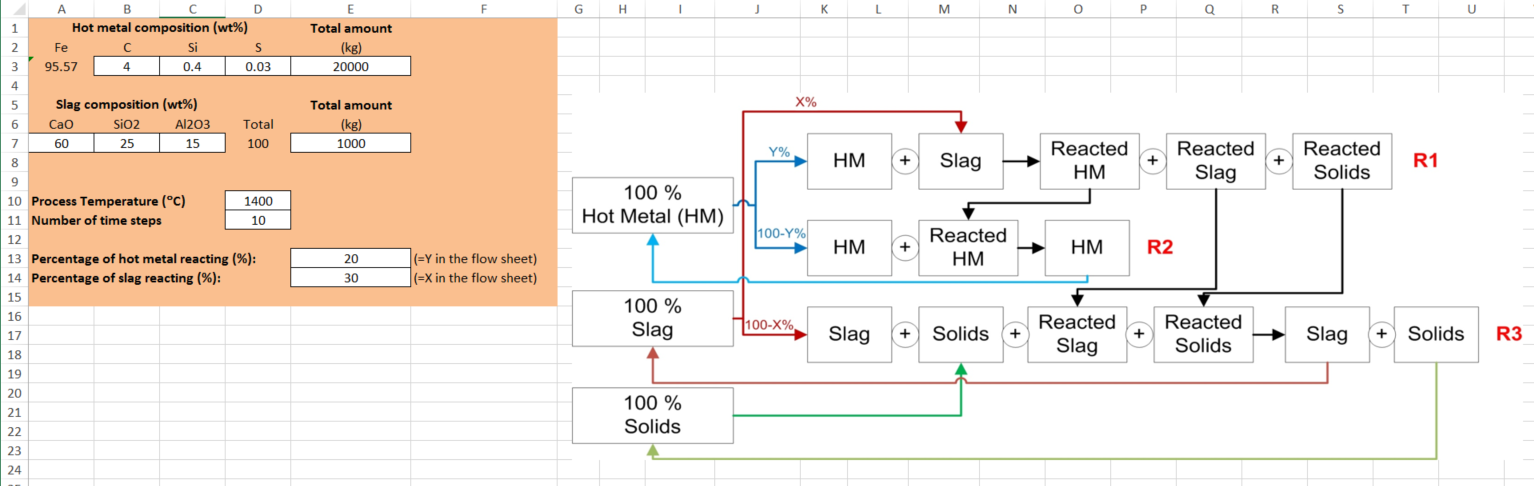The process is broken down into 3 reactions: A fraction of the hot metal is equilibrated with a fraction of the slag in R1 In R2, the hot metal product from R1 is mixed back with the remaining hot metal In R3, the slag products from R1 (including liquid slag and pure compounds) are mixed with the remaining top slag Each phase (i.e. hot metal, liquid slag and pure compounds) is saved in a [stream] and passed to the next Equilib file or to the next time step Reactions R1, R2 and R3 are repeated at each time step, until the total process duration is reached.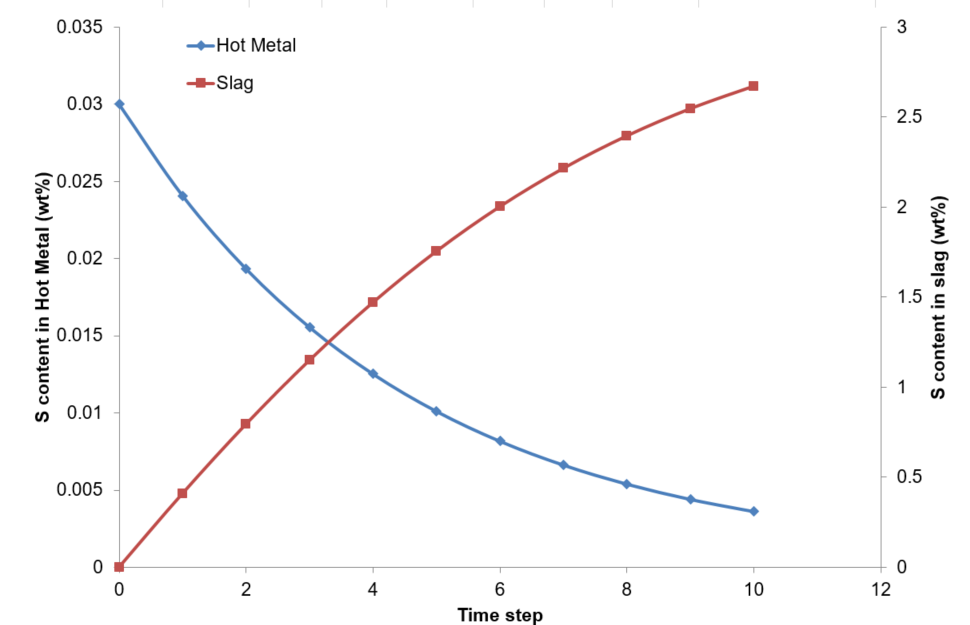FactSage 7.2 (2018)

 PhasEx_Superimpose_Figs.mac is a new macro file in FactSage 7.2. This Phase Diagram macro is particularly useful if you wish to superimpose several calculated phase diagrams into one figure. . Copy PhasEx_Superimpose_Figs.mac to the folder that contains the *.fig files of the type MyName-1.fig, MyName-2.fig, MyName-3.fig ... Run 'Phase Diagram' and then 'Phase Diagram - Components Window > File > Macro Processing > Run .... PhasEx_Superimpose_Figs.mac'. The macro: asks you to enter the common name of the fig files - e.g. MyName- loads each MyName-*.fig and superimposes it with the others. displays the superimposed figure saves the superimposed figure in MyName-Superimposed.fig The screenshot shows four Ag-Au-Bi isothermal sections superimposed into one figure.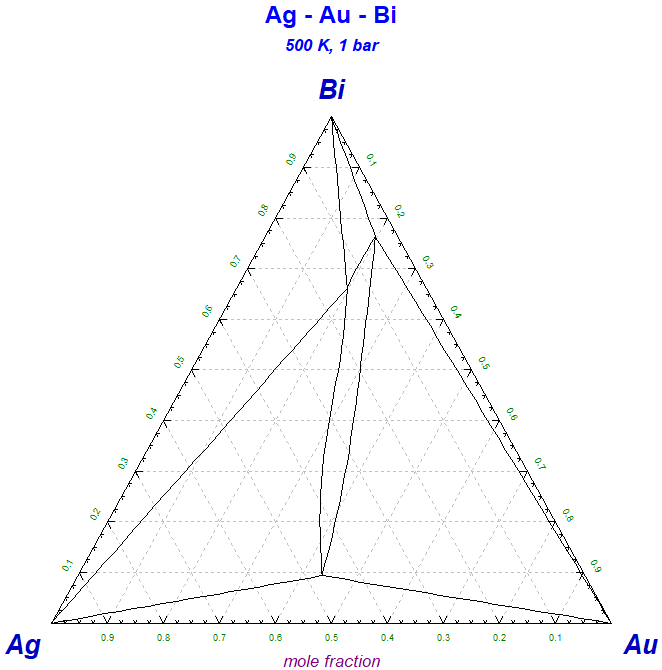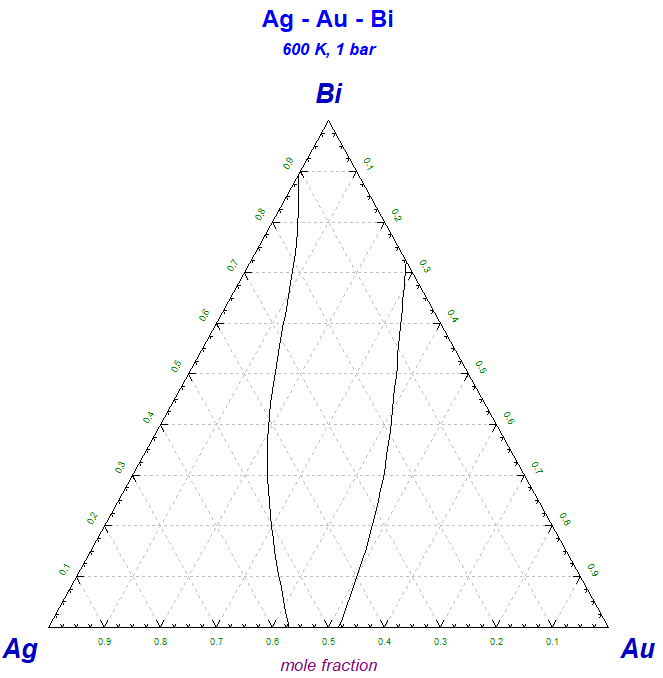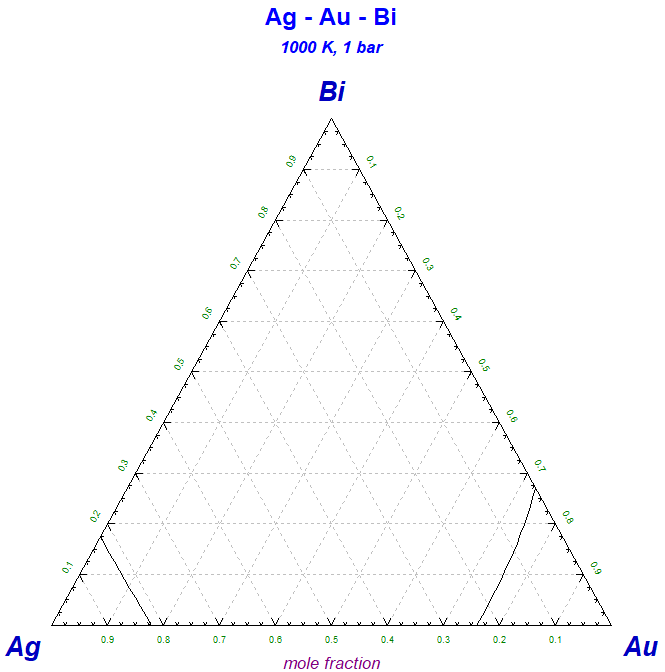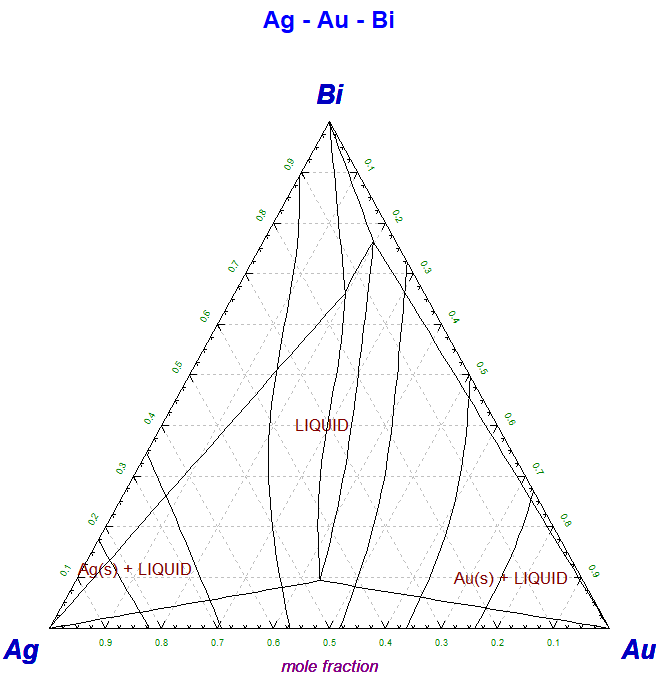.

 PhasEx_Calc_All_PDs.mac is a new macro file in FactSage 7.2. This Phase Diagram macro is particularly useful for database developers who wish to recalculate phase diagrams using revised databases. The macro loads each Phas*.dat that is stored in a folder, calculates the phase diagram and then displays the calculated figures in a web browser (htm) format. If the Phas*.dat contains a stored diagram it is displayed as well. In this manner the developer can compare the newly calculated diagram with the old one. Copy PhasEx_Calc_All_PDs.mac to the folder where the Phas*.dat files are stored. Run 'Phase Diagram' and then 'Phase Diagram - Components Window > File > Macro Processing > Run .... PhasEx_Calc_All_PDs.MAC'. The macro: loads each Phas*.dat file and calculates the phase diagram the calculated phase diagram is stored in the \BMPs\ folder as a bmp file both the calculated diagram and the previously saved figure are displayed side by side in an htm file (Phas_Calc_All_PDS.htm) as shown in the screenshot the elapsed time to calculate the phase diagram and the total accumulated time are posted In order to permanently save a record of all calculated and saved figures use 'Word' to open Phas_Calc_All_PDS.htm and save as a *.pdf file - for example Phas_Calc_All_PDS.pdf.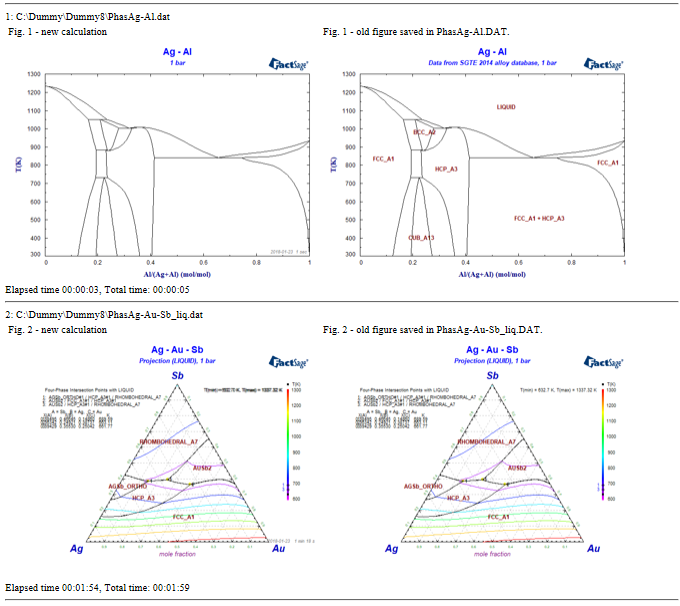.

FactSage 7.3 (2019)

FactSage 8.0 (2020)

• Macros and the new file naming convention - *.equi, *.phas, *.mixt

In FactSage 8.0 private files PROG*.dat have been replaced by *.prog files.

The macro function \$EXIST() and the macro commands OPEN and SAVE have been modified and are backward compatible it is not necessary to apply the new naming convention to old *.mac files. That is, you can load and run old *.mac files and not worry about the new naming convention.

\$EXIST()

```
\$EXIST('FileName')        // = 1 if 'FileName' exists
\$EXIST(EQUIEx_CH4-O2.dat) // FactSage 7.3 (and prior) naming convention
\$EXIST(Ex_CH4-O2.equi)    // FactSage 8.0 naming convention
```
The macro function \$EXIST is backward compatible.
For example, \$EXIST(EQUIEx_CH4-O2.dat) returns a value 1 if the file Ex_CH4-O2.equi exists.

OPEN

```
OPEN 'FileName'        // open (load) Equilib and Phase Diagram files
OPEN EQUIEx_CH4-O2.dat // FactSage 7.3 (and prior) naming convention
OPEN Ex_CH4-O2.equi    // FactSage 8.0 naming convention
```
The macro command OPEN is backward compatible.
For example the command OPEN EQUIEx_CH4-O2.dat opens the file Ex_CH4-O2.equi if it exists.

SAVE Run macro menu - 'Equilib > Reactants Window > Run Macro ...'

 'Run Macro' has been added to the Menu of the Equilib Reactants Window. This enables you to immediately rerun the last macro that was loaded.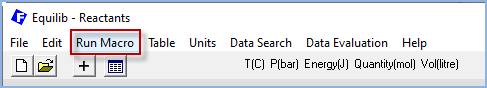The 'Run Macro' menu button is equivalent to 'Equilib > Reactants Window > File > Macro Processing > Run macro ...'

> SAVE 'Mixture' GAS (LIQUIDS SOLIDS SOLUTIONS 'phase-name') // save results in a stream SAVE MIXTMyGas.dat GAS // FactSage 7.3 (and prior) naming convention SAVE MyGas.mixt GAS // FactSage 8.0 naming convention The macro command SAVE 'Mixture' is backward compatible.
For example SAVE MIXTMyGas.dat saves the mixture in MyGas.mixt.

Macro example

For an example of backward compatibility run the macro EquiEx_SET_Variables.mac located in the Macros Directory.

The macro log file posts '-------> Backward compatible: ...' each time the issue is encountered.

• Encrypted macros

In FactSage 8.0 it is now possible to encrypt a macro file (*.mac) and create an encrypted macro file (*.mace).  To perform the encryption go to the Equilb Reactants Window. 1. Click 'File > Macro processing ... > Encrypt macro ...' and open the macro file (*.mac) you wish to encrypt. 2. The resulting encrypted macro file (*.mace) will be stored in the same location as the source file. 3. Note there is no option to decipher the encrypted macro.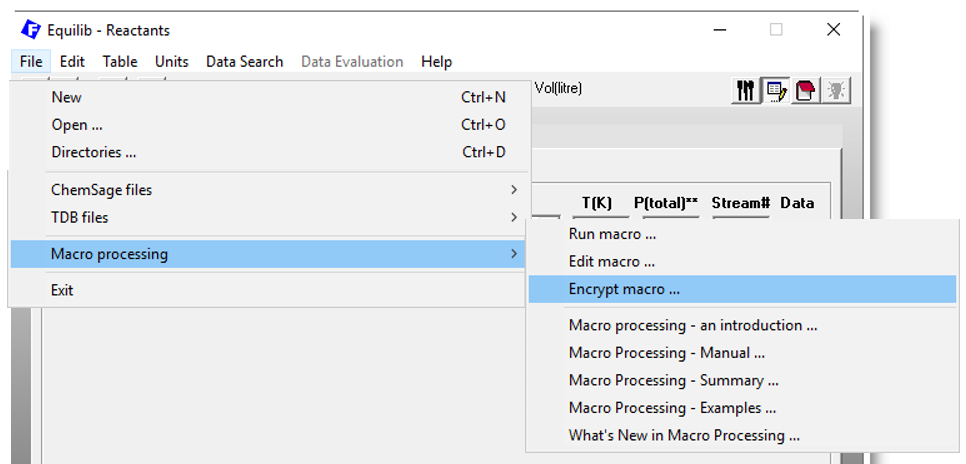The advantages of an encrypted macro file are:

• Security. The encrypted file cannot be modified or edited. Any attempts to do so render the file corrupt and useless.

• Confidentiality. The contents of the original macro source file are hidden. A user cannot see the methodology employed in the macro processing.

When the macro processing is run the lines of code are displayed in real time in the Macros Window. Use the macros command PRINTOFF to suppress the output and hide the code. Use PRINTON to reactivate the output. See the example below for the commands PRINTOFF and PRINTON.

• Mobility. The encrypted file is a simple text file that can be copied and emailed as an attachment.

The encrypted macro file (*.mace) is employed in macro processing in the same manner as a normal macro file (*.mac).

The macro functions \$USERID and \$USERNAME provide the user ID (1-9999) and company name or affiliation.

Let us say you wish to give macro access only to customer ID 123 Company A-B-C.
Insert the following commands into the macro file.  ``` DIM %ID %NAME %ID = \$USERID %NAME = \$USERNAME PRINTOFF // The following lines will NOT appear in the Macros Window. IF %ID <> 123 THEN // i.e. if ID is not 123 %TITLE = User ID %ID %NAME MSGBOX Access limited to ID 123 - Company ABC GOTO END // terminate the macro processing ENDIF PRINTON // The following lines will appear in the Macros Window. ... ```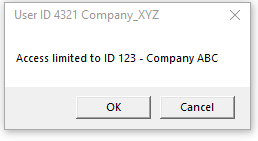```IF %ID <> 123 AND %ID <> 456 AND %ID <> 789 THEN
```
```IF %ID < 200 OR %ID > 300 THEN
```
When finished remember to encrypt the macro file.

FactSage 8.1 (2021)

• Run macro menu - 'Equilib > Reactants Window > Run Macro ...'

 'Run Macro' has been added to the Menu of the Equilib Reactants Window. This enables you to immediately rerun the last macro that was loaded.The 'Run Macro' menu button is equivalent to 'Equilib > Reactants Window > File > Macro Processing > Run macro ...'

• What's New in Macro Processing - 'Equilib > Reactants Window > File > Macro Processing > What's New in Macro Processing ...'
```
MACRO_SHOW   [TOP BOTTOM LEFT RIGHT]
- show the macro window (also see MACRO_HIDE)
- MACRO_SHOW TOP - place macro window at the top center
- MACRO_SHOW BOTTOM RIGHT - place macro window at the bottom right corner. New in 8.1

SET PARAMETERS TIME_CALC_SECS_LIMIT  '0 - 86400'
- stop the calculation after TIME_CALC_SECS_LIMIT exceeded
- this redefines the 'time limit' posted in the STOP Window

\$SOLGAS - compilator and date ex: "Lahey_2020-12-18" "Intel_2020-12-18"
- used by FactSage developers, new in 8.1

Species:
- \$E_s?*\$ - product species * = 1 - 5000; ? = a, D, d, E, f, g, h, l, N, n, R, s, W, w, X ...
- these values are only defined after the equilibrium has been calculated (CALC) i.e. \$E_OK\$ = 'OK'

\$E_so*\$ = species "o" flag in Equilib Results; assumed to have zero molar volume (1=True, 0=False)
\$E_sT*\$ = species "T" flag in Equilib Results; data are extrapolated above maximum T limit (1=True, 0=False)
\$E_sV*\$ = species "V" flag in Equilib Results; modeled with an equation of state (1=True, 0=False)

Examples of "o" "T" "V" flags are given in the updated EquiEx_List_Results.mac
located in the Macros Folder.

Elements:  - atomic numbers expanded to 132 (was 99)
- updated \$ELEMENT_NAME('atomic number') \$ELEMENT_NUMBER('name')
- new \$ELEMENT_NAME-FULL('atomic number') \$ELEMENT_NUMBER_MAX
for up to 132 elements including "virtual elements".

Example is given in the new EquiEx_List_Elements.mac located in the Macros Folder.
```

[www.FactSage.com] [CRCT] [GTT]